# A Nonstationary Mathematical Model for Acceleration Time Series

A Nonstationary Mathematical Model for Acceleration Time Series
Ecole Normale Supérieure (ENS-Kouba) Algeria, Laboratory: Mathematical Physics and Applications (LPMA), Kouba 16308, Algeria

Faculty of Sciences, Amar TELIDJI University, UAT, Laghouat 03000, Algeria

Materials Physics and Chemistry Laboratory, Amar TELIDJI University, UAT, Laghouat 03000, Algeria

Corresponding Author Email:
t.brahimi@ens_lagh.dz
Page:
246-252
|
DOI:
https://doi.org/10.18280/mmep.080211
3 August 2020
|
Accepted:
16 December 2020
|
Published:
28 April 2021
| Citation

OPEN ACCESS

Abstract:

The choice of nonstationary stochastic models for the study is fully justified by the limitation of acceleration time series number. The three acceleration time series under consideration are used to generate a new, artificial series of ten per historical one using autoregressive moving average model. Subsequently, the average of nonlinear is utilized for the ten acceleration time series in order to obtain the spectral response of a system with single degree of freedom.

Modeling of acceleration time series involves critical estimation of metrics that characterize nonstationary acceleration time series. Thus, for the stiffness degrading systems and bilinear systems, the metrics of hysteretic energy demand and displacement ductility demand during displacement are used.

The applicability of artificially generated acceleration time series for the qualitative description of information was shown. More specifically, ARMA (2,2) showed the best results in the study for three accelerated time series through nonlinear response analysis. In addition, as a result, normalized hysteretic energy demand, empirically valid displacement ductility relationships, and model parameters were proposed.

Keywords:

autoregressive, nonstationary, stochastic, ductility, hysteretic

1. Introduction

Both time and frequency domains are utilized to generate acceleration time series from stochastic models. Extensive reviews of acceleration time series process models in the frequency and times domain have been presented by Kozin ; Shinozuka et al.  and Chang et al. . A number of papers in the literature have reported ARMA models. ARMA models are discussed in detail by Box and Jenkins . The basic concept of using artificial acceleration in the seismic analysis was proposed by Housner and Jennings  and Jack , and other researchers have studied the correlation between model parameters [7, 8].

2. ARMA Models

The autoregressive moving average ARMA models at any time step “t” may be represented as follows:

$Z_{t}-\varphi_{1} Z_{t-1}-\cdots-\varphi_{p} Z_{t-p}=W_{t}-\theta_{1} W_{t-1}-\cdots-\theta_{q} W_{t-q}$    (1)

where, $\varphi_{i}$, $\theta_{j}$ are constant coefficients.

The Eq. (1)'s left side represents the autoregressive, AR, part of the order “p.” Thus, the measured data sequence used is time series [$Z_{k}$]. Meanwhile, the Eq. (1)'s right side as part of order “q” is the moving average, MA. Then, the sequence [$W_{t}$] is a set of identically distributed and independent Gaussian variables.

It must be said that the digitized data $A_{t}$ is first normalized in this study. Estimating the variance or modulating function is a critical problem in modeling acceleration time series since it controls the non-stationarity of the process and statistical parameters, such as the extreme values of acceleration and structural response. The root mean square of $S_{t}$ is calculated for a moving window of hundredfold steps centered on time step “t.” The given acceleration time series is then normalized to obtain a stationary acceleration time series, $\left[Z_{t}\right]$, with zero mean and unit variance.

$\left[Z_{t}\right]=\left[A_{t}\right] /\left[S_{t}\right]$    (2)

Hence, $\left[Z_{t}\right]$ is considered as a stationary process of the Eq. (1).

Referring to the pattern of the partial autocorrelation and autocorrelation functions is a necessary step to clarify information about the ARMA process's order selection (p,q).

Estimation of the number of parameters and their numerical values to fit a model to a time series is a basic problem. The estimation of parameters is based on the nonlinear least squares, but the order of the model is based on the Akaike  Information criterion.

3. Application of ARMA Models for Acceleration Time Series

3.1 Data

In this study, the data consist of three acceleration time series were measured: Afroun with 16000 data points digitized at 0.005 seconds, Ain Defla with 5000 data points digitized at 0.005 seconds, and Dar Beida with 5528 data points digitized at 0.005 seconds. Shown in Figure 1, Figure 2, Figure 3 are plots of the measured acceleration time series.

## 1.pngFigure 1. Afroun acceleration time series

## 2.png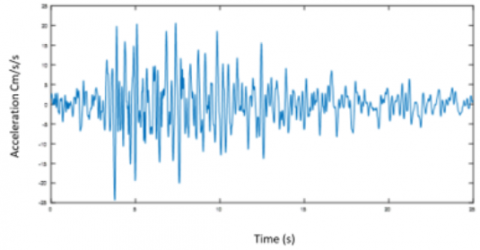Figure 2. Ain defla acceleration time series

## 3.png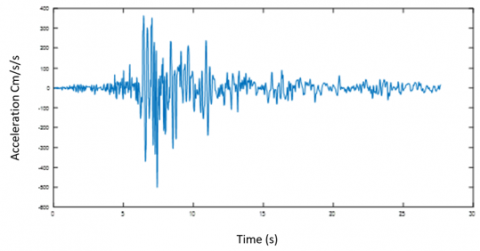Figure 3. Dar El beida acceleration time series

3.2 Modeling procedure

The following five-step algorithm must be performed when installing the ARMA model in an acceleration time series:

1. Calculate the experimental modulating function $S_{t}$ and normalize the given acceleration time series.

2. Suppose a simple general analytical form f(t) to fit the modulating function $S_{t}$, and estimate the parameters $\alpha, \beta$, and $\gamma$.

$f(t)=\alpha e^{-\left(\frac{t-\beta}{\gamma}\right)^{2}}$    (3)

Shown in Figure 4, Figure 5, and Figure 6 are the modulating and envelope functions for the three measured acceleration time series.

1. Select the order (p,q) based on the partial autocorrelation and autocorrelation and functions.

2. Estimate the coefficients $\varphi_{i}$, i=1, 2…, p and $\theta_{j}, j=1,2, \ldots, q$.

3. Select the model order based on the AIC (p,q) criterion.

It must be said that to perform steps 3 and 4 it is necessary to apply STATGRAPHICS . The ARMA parameters and envelope function parameters are shown in Table 1.

Table 1. ARMA and envelope function parameters

 ARMA & Envelope Function Parameters Afroun Ain Defla Dar Beida $\varphi_{1}$ 1.8635 0.944 1.9080 $\varphi_{2}$ -0.922845 -0.963 -0.9590 $\theta_{1}$ -1.28836 -0.469 -1.1503 $\theta_{2}$ -0.954263 -0.295 -0.8881 $\sigma_{w}$ 0.0407843 0.0210 0.03357 α 27.36 6.081 126.2 β 18.26 8.098 8.130 γ 3.290 8.991 3.290

## 4.png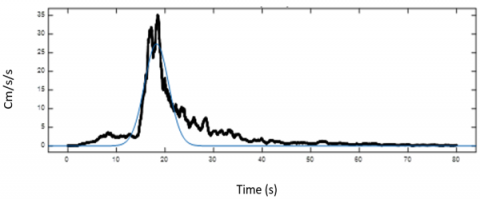Figure 4. Afroun measured and envelope functions

## 5.png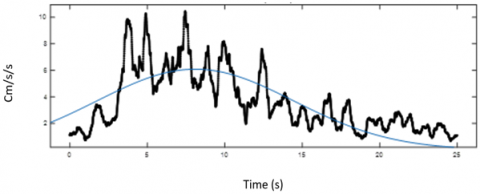Figure 5. Ain defla measured and envelope function

## 6.png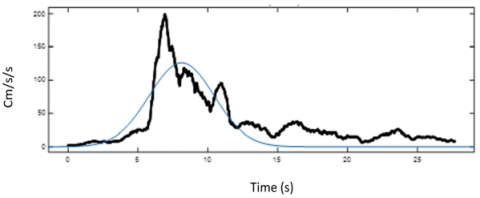Figure 6. Dar beida measured and envelope functions

3.3 Acceleration time series simulation

To simulate acceleration time series, stationary time series are first generated using the fitted ARMA model and then multiplied by the fitted parametric envelope function Eq. (3). Since ARMA model is a linear combination of past values of  $Z_{t}$ and Gaussian values $W_{t}$, simulated time series can be generated recursively. $W_{t}$ are Gaussian random variables with zero mean and variance $\sigma_{w}^{2}$. Shown in Figure 7, 8, and 9, are simulated acceleration times series from the ARMA (2,2) models as fitted to Ain Defla, Dar Beida, and Afroun acceleration time series.

## 7.png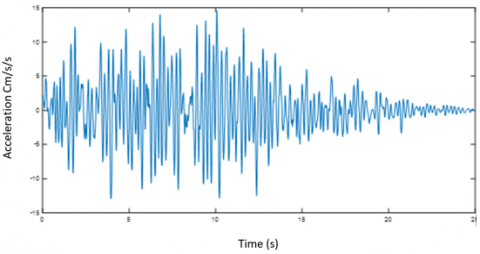Figure 7. Ain defla simulated acceleration time series

## 8.png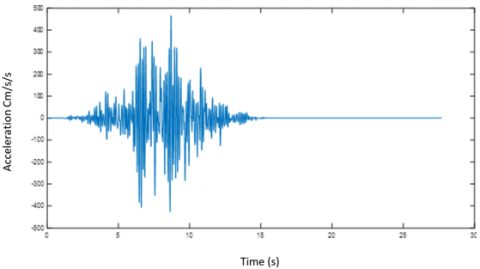Figure 8. Dar beida simulated acceleration time series

## 9.png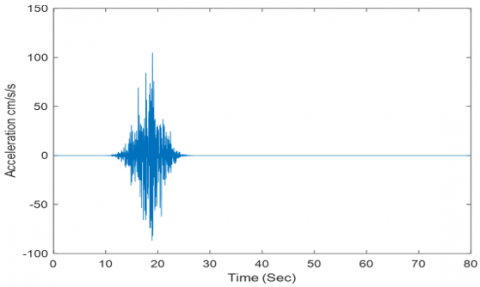Figure 9. Afroun simulated acceleration time series

4. Response Spectra

The ARMA models provide an effective means in characterizing the non-stationarity of records which can be used as input in structural analysis. Each acceleration time series is an output time-series event from a representative class of stochastic processes characterized by the ARMA models.

Structural response spectra provide an excellent means to describe a ground acceleration time series. A single degree of freedom system with viscous damping is used to obtain the spectral response. Figure 10. System stiffness can be bilinear or stiffness degrading. Then, it is necessary to apply a stepwise numerical integration of the general equation that considers the linear acceleration at each time step . This strategy is used to obtain the spectral response for a unique acceleration time series. The equation is:

$M \ddot{U}(t)+c \dot{U}(t)+R(U, t)=-M \ddot{U}_{g}(t)$    (4)

where, U(t) is the relative mass displacement for the ground, c is the damping coefficient, M is the mass, and $\ddot{U}_{g}(t)$ is the acceleration of M relative to the fixed reference axis, and R(U,t) is the restoring force.

## 10.png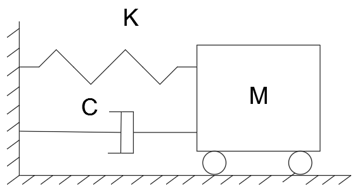Figure 10. Single degree of freedom system

5. Damage Measures

Several measures have been proposed by a number of investigators. These damage measures are expressed as functions of structural response parameters to summarize the effect of acceleration time series on linear and no-linear systems. A comparative of damage measures study was made by Grigoriu , the implementation of these arrangements has been carried out by Pappas et al.  and Chopra .

5.1 Maximum displacement

For design purposes, it is generally essential to know the maximum absolute value of the response subjected to an acceleration time series.

$U_{\max }=\max |U(t)|$    (5)

This dependence function, reflecting the relationship between the maximum value and the period or frequency of natural vibration, provides a classical spectrum for systems with the same damping value and periodic range.

5.2 Maximum displacement ductility

If the maximum absolute value of the displacement response calculated at its full excitation is divided by the value of the yield displacement of the system, it becomes possible to obtain the maximum displacement ductility realized as a normalized value. Thus, the maximum displacement ductility μ less than one indicates an elastic response.

5.3 Normalized hysteretic energy

If the amount of energy expended by the system to dissipate in the case of full excitation is divided by twice the energy absorbed at the first yield plus one, it is possible to obtain hysteretic energy, defined as the normalized value. The energy dissipated in a structure with the hysteretic load-deformation relationship is given by

$E_{h}(t)=\int_{0}^{t} R(u, t) \dot{u}(t) d t-E_{s}(t)$    (6)

where, $E_{S}$ is the elastic strain energy given by:

$E_{S}=\frac{1}{2} K_{y} U_{y}^{2}$    (7)

R(u,t) is the restoring force $d u=\dot{u}(t) d t$.

The normalized hysteretic energy will be then

$E_{N H}=1.0+\frac{E_{H}}{R_{y} U_{y}}$    (8)

where, $R_{y}=K_{y} U_{y}$ is the yield force.

6. Applications and Results

Multiple ARMA models were successfully used to analyze the three acceleration time series, with empirical data recorded for further processing. For ease of visualization, information on the projected ARMA parameters and envelope functions are summarized in Table 1. Consequently, the following steps were used to build the models. First, a sample of ten artificial acceleration time series was generated for each parameter set of the three natural series, and as illustrated in Figures 7, 8, and 9, even the simple model generally described well the periodicity of events peculiar to the Afroun, Ain Defla,  and Dar Beida acceleration series.

Then, the damping ratio numerical values ε=0.05 with yield ratio $Y=R_{Y} / M g$ of 0.05, 0.1, 0.15, 0.2, 0.3 were used for the response analysis. Other yield ratios were used for the hysteretic energy Y = 0.01, 0.02, 0.03, 0.04.

Subsequently, the hysteretic energy demand spectra coupled with the mean displacement ductility were calculated for the three acceleration time series. To establish a confidence interval, the standard deviation was computed for each output event. The results are shown in Figure 11. In addition, the results were obtained using bilinear and stiffness degrading systems.

As a general remark, the spectra ordinates decrease with an increase in the period. The mean response spectra show a stable and smooth curve with changing frequencies. Spectral ordinates for both stiffness degrading systems and bilinear ones are in general similar spectral shapes.

Since the inelastic response is affected by the initial yield displacement, the yield strength ratio, which provides the initial yield displacement, is the most critical factor in the analysis of nonlinear systems.

## 11.png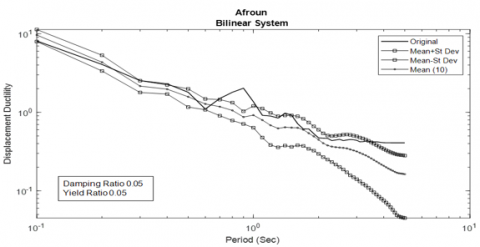(a)

## 12.png(b)

## 13.png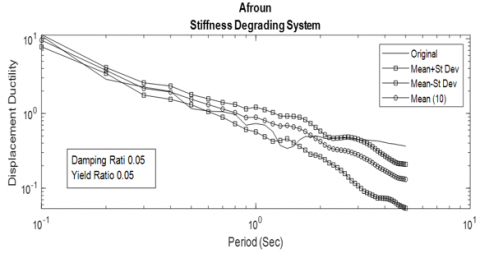(c)

## 14.png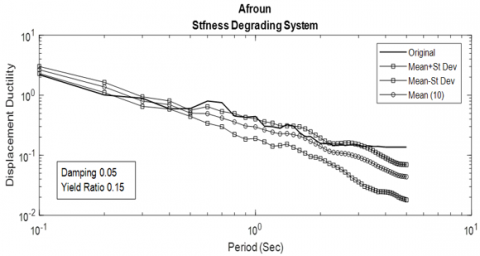(d)

## 15.png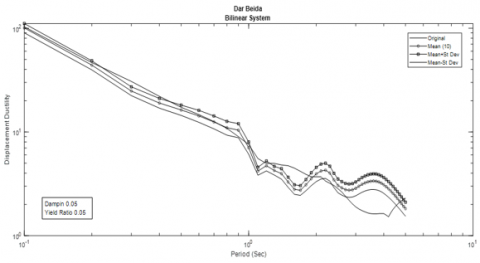(e)

## 16.png(f)

## 17.png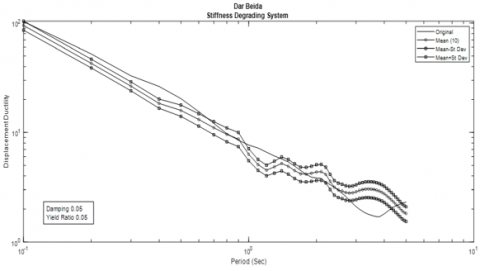(g)

## 18.png(h)

## 19.png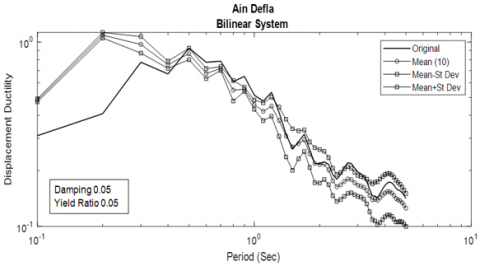(i)

## 20.png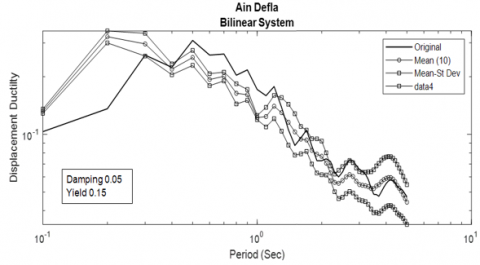(j)

## 21.png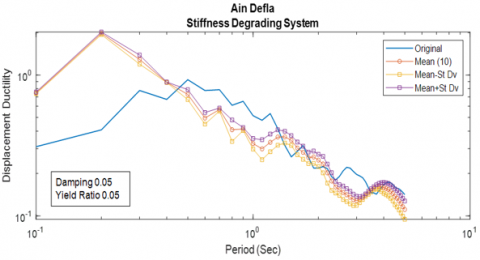(k)

## 22.png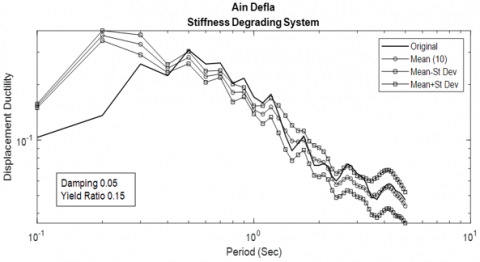(l)

Figure 11. One confidence intervals for displacement ductility

## 23.png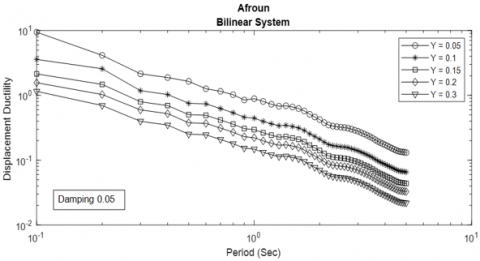(a)

## 24.png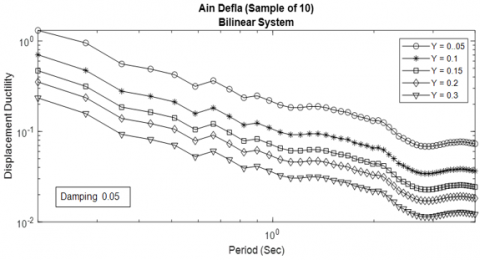(b)

## 25.png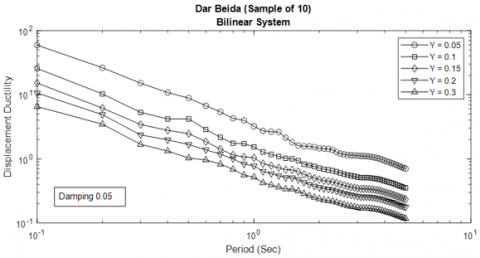(c)

Figure 12. Effect of yield strength ratio (Sample of 10)

Figure 12 shows typical examples of displacement ductility for yield ratios of 0.05, 0.1, 0.15, 0.2, 0.3, assuming 5% damping for a bilinear system. Figure 13 shows examples of Afroun, Ain Defla, and Dar Beida using same yield ratios and damping.

## 26.png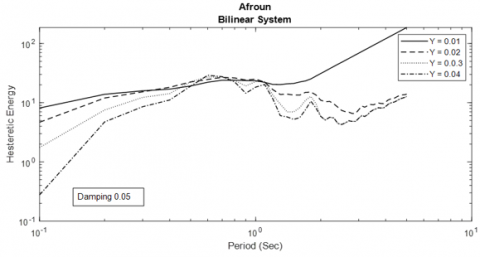(a)

## 27.png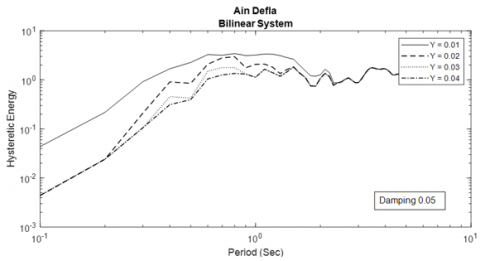(b)

## 28.png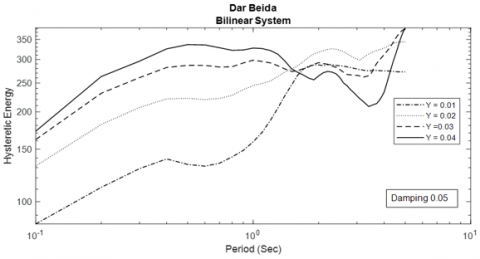(c)

Figure 13. Effect of yield strength ratio

Several useful patterns emerged from this analysis. First of all, the results obtained for the spectral response for the two quantities showed that the logarithm of the average nonlinear response demand spectrum was linearly related to the logarithm of the natural period under certain conditions. Then, based on the empirical relationships for the normalized hysteretic energy demand spectra $E_{H}$ and for the displacement ductility μ, the following relationships can be inferred:

$\log (\mu)=c_{1}+c_{2} \log (T)$    (9)

$\log \left(E_{H}\right)=c_{3}+c_{4} \log (T)$    (10)

where, $c_{1}$, $c_{2}$, $c_{3}$, $c_{4}$ are constants related to Model and system parameters, and T natural period.

7. Conclusions

(1) In fact, the application of the time-domain approach using ARMA models provides uncomplicated results for a limited number of parameters, while practical implementation requires imposing restrictions on the number of model parameters.

(2) A good description of the average spectral response can be derived from the assumption that the acceleration time-series event is one of the sampling realizations for the set of such time series corresponding to the baseline event

(3) A generally linear relationship was also found between the logarithm of the average spectra of the nonlinear response of the two quantities and the logarithm of the system's natural period.

(4) For a given system period and damping, the response spectra (displacement ductility and Hysteretic energy) decrease when the logarithm of the system period increases.

References

 Kozin, F. (1988). Autoregressive moving average models of earthquake records. Probabilistic Engineering Mechanics, 3(2): 58-63. https://doi.org/10.1016/0266-8920(88)90016-1

 Shinozuka, M., Deodatis, G., Harada, T. (1987). Digital simulation of seismic ground motion. In Stochastic Approaches in Earthquake Engineering, pp. 252-298. https://doi.org/10.1007/978-3-642-83252-9_14

 Chang, M.K., Kwiatkowski, J.W., Nau, R.F., Oliver, R.M., Pister, K.S. (1982). ARMA models for earthquake ground motions. Earthquake Engineering & Structural Dynamics, 10(5): 651-662. https://doi.org/10.1002/eqe.4290100503

 Box, G.E.P., Jenkins, G.M., Gregory, C.R. (2008). Time Series Analysis Forecasting and Control. Fourth Edition, New Jersey, Wiley.

 Housner, G.W., Jennings, P.C. (1964). Generation of artificial earthquakes. Journal of the Engineering Mechanics Division, 90(1): 113-150. https://doi.org/10.1061/JMCEA3.0000448

 Baker, J.W. (2011). Conditional mean spectrum: Tool for ground-motion selection. Journal of Structural Engineering, 137(3): 322-331. https://doi.org/10.1061/(ASCE)ST.1943-541X.0000215

 Basse-O'Connor, A., Nielsen, M.S., Pedersen, J., Rohde, V. (2020). Stochastic delay differential equations and related autoregressive models. Stochastics, 92(3): 454-477. https://doi.org/10.1080/17442508.2019.1635601

 Akaike, H. (1974). A new look at the statistical model identification. IEEE T. Automat. Contr., 19: 716-723. https://doi.org/10.1109/TAC.1974.1100705

 STATGRAPHICS 18. (2015). Software program, Copyright 2015 by Statpoint Technology, Inc.

 Boore, D.M., Atkinson, G.M. (1987). Stochastic prediction of ground motion and spectral response parameters at hard-rock sites in eastern North America. Bulletin of the Seismological Society of America, 77(2): 440-467. https://doi.org/10.1016/0040-1951(87)90116-8

 Grigoriu, M. (1987). Damage Models for Seismic Analysis. Dep. Of Civil Eng., Cornell University.

 Pappas, S.S., Ekonomou, L., Karamousantas, D.C., Chatzarakis, G.E., Katsikas, S.K., Liatsis, P. (2008). Electricity demand loads modeling using AutoRegressive Moving Average (ARMA) models. Energy, 33(9): 1353-1360. https://doi.org/10.1016/j.energy.2008.05.008

 Chopra, A.K. (2017). Dynamics of Structures 5th Edition. University of California at Berkley, Pearson.